Got Feedback?

Found a bug? Have a suggestion? Fill the form below and we'll take a look!

X

# Discriminant Calculator

The Discriminant tells about the nature of the roots of quadratic equation. In quadratic equation formula, we have $$b^2 - 4ac$$ under root, this is discriminant of quadratic equations.
There are three cases for discriminant:
if $$b^2 - 4ac = 0$$, then the roots of quadratic equations are real and equal.
if $$b^2 - 4ac > 0$$, then the roots are real but not equal
if $$b^2 - 4ac < 0$$, then the roots are not equal and are imaginary.

$$a x^2 + bx + c = 0$$

## What is Discriminant Algebra?

When solving with the quadratic formula the “discriminant” is the value that helps us figure out the types of solution. It is a special functions of the co-efficient of an equation (quadratic equation) whose value reveals information about the roots of the equation.

The discriminant is calculated by squaring the “b” term and subtraction 4-times the “a” term times the “c” term. Discriminant is also represented by Δ (delta) beside “D”.

## Discriminant Formula

The expression of the form $$b^2 – 4ac$$ is called discriminant of the quadratic equation.
$$ax^2 + bx + c = 0; a ≠ 0$$

## Standard Form

Roots of quadratic equation $$x = \dfrac{-b \pm \sqrt{b^2 – 4ac}}{2a}$$

Here, discriminant is $$b^2 – 4ac$$ and it is used to find the nature of the roots besides number of the roots. It is represented by D or Δ.

## What does a positive and negative discriminant represent?

A positive discriminant denotes that the quadratic has two different real numbers solutions. A discriminant of zero denotes that the quadratic consist of a repeated real number solution. A negative discriminant denotes that neither of the solution is real number.

## How do you find the discriminant of quadratic equation?

$$D > 0$$ (2 real solutions)
$$D = 0$$ (1 real solution)
$$D < 0$$ (2 imaginary solutions)

If $$D > 0$$ then two real solutions
1- If perfect square; 2 rational roots
2- If not perfect square; 2 irrational roots
N.B: real solutions occur when the graph hits the x-axis.

Example no 1:
Let $$x^2 – 4x + 2 = 0$$

Solution:
By substitution respective values as $$a = 1$$, $$b = -4$$, $$c = 2$$ in equation.
$$x = \dfrac{4 \pm \sqrt{(-4)^2 – 4(1)(2)}}{2(1)}$$
$$x = \dfrac{4 \pm 2\sqrt{2}}{2}$$
$$x = 3.4$$ or $$x = 0.59$$

Now $$D = b^2 – 4ac$$ By substitution respective values as $$a = 1$$, $$b = -4$$, $$c = 2$$ in equation.
$$D = (-4)^2 – 4(1)(2) = 16 – 8 = 8$$

As $$D > 0$$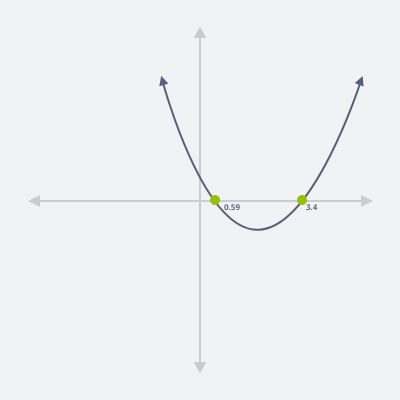Example no 2:
Let $$x^2 – 4x + 4 = 0$$

Solution:
By substitution respective values as $$a = 1$$, $$b = -4$$, $$c = 4$$ in equation.
$$x = \dfrac{4 \pm \sqrt{(-4)^2 – 4(1)(4)}}{2(1)}$$
$$x = \dfrac{4 \pm \sqrt{16 – 16}}{2}$$
$$x = \dfrac{4 \pm 0}{2}$$
$$x = \dfrac{4}{2}$$
$$x = 2$$

Now $$D = b^2 – 4ac$$ By substitution respective values as $$a = 1$$, $$b = -4$$, $$c = 4$$ in equation.
$$D = (-4)^2 – 4(1)(4) = 16 – 16 = 0$$

As $$D = 0$$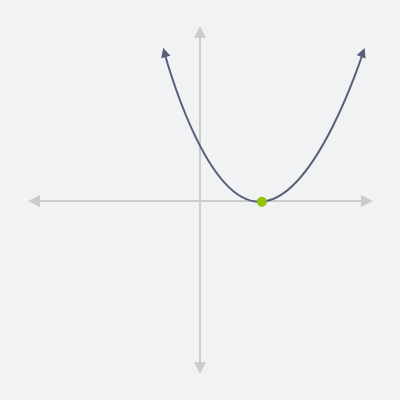Example no 3:
Let $$x^2 – 4x + 13 = 0$$

Solution:
By substitution respective values as $$a = 1$$, $$b = -4$$, $$c = 13$$ in equation.
$$x = \dfrac{4 \pm \sqrt{(-4)^2 – 4(1)(13)}}{2(1)}$$
$$x = \dfrac{4 \pm \sqrt{16 – 52}}{2}$$
$$x = \dfrac{4 \pm \sqrt{-36}}{2}$$
$$x = \dfrac{4 \pm 6i}{2}$$
$$x = 2 \pm 3i$$

Now $$D = b^2 – 4ac$$ By substitution respective values as $$a = 1$$, $$b = -4$$, $$c = 13$$ in equation.
$$D = (-4)^2 – 4(1)(13) = 16 – 52 = -36$$

As $$D < 0$$, so this equation has 2 imaginary solutions. By putting the values of x in equation
$$(2 \pm 3i)^2 – 4(2 \pm 3i) + 13 = 0$$
$$4 + 12i + 9i – 8 – 12i + 13 = 0$$
As $$i^2 = -1$$
$$4 + 12i + 9(-1) – 8 – 12i + 13 = 0$$
$$4 – 9 – 8 + 13 = 0$$
$$0 = 0$$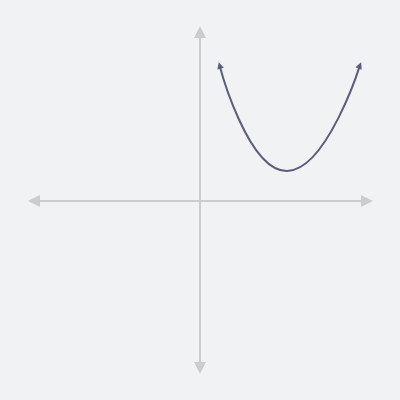Q: Find out the number of solutions the given equation has by using its discriminant. Check whether the solutions are real or imaginary?

Step no 1: $$x^2 + 3x – 8 = 0$$ (take a quadratic equation)

Step no 2: Compare the equation with standard form $$ax^2 + bx + c = 0$$ to get the values of a, b and c.

Step no 3: Find discriminant Δ

$$Δ = b^2 – 4ac = (3)^2 – 4(1)(8) = 9 + 32 = 41$$

As $$Δ > 0$$, so it has two real solution.

Step no 4: Simplify quadratic equation for making graph.

## What does the $$b^2 – 4ac$$ shows?

The expression $$b^2 – 4ac$$ is called the discriminant, which determines the actual number of a exact solutions.

## Discriminant of a quadratic equation

Consider a quadratic equation, $$Y = ax^2 + bx + c$$. The discriminant $$D = b^2 – 4ac$$ define the types of the roots of the specific equation has,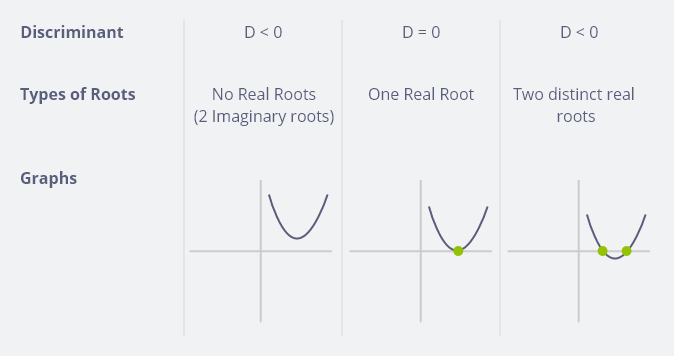## Examples of discriminant calculation

Example 1: $$9x^2 – 12x + 4 = 0$$
For D: $$Δ = b^2 – 4ac = (-12)^2 – 4(9)(4) = 144 – 144 = 0$$. As discriminant is zero, so it has one real root.

Example 2: $$-4x^2 – 12x – 9 = 0$$
For D: $$Δ = b^2 – 4ac = (12)^2 – 4(-4)(-9) = 144 – 144 = 0$$. As discriminant is zero, so it has one real root.

Example 3: $$x^2 – 2x + 3 = 0$$
For D: $$Δ = b^2 – 4ac = (-2)^2 – 4(1)(3) = 4 – 12 = -8$$. As discriminant is negative, so it has no real solution.

Example 4: $$x^2 + 2x – 5 = 0$$
For D: $$Δ = b^2 – 4ac = (2)^2 – 4(1)(-5) = 4 + 20 = 24$$. As discriminant is positive, so it has two real solution.

## Quick review

The discriminant $$b^2 – 4ac$$

If $$D > 0$$ -> Shows 2 real roots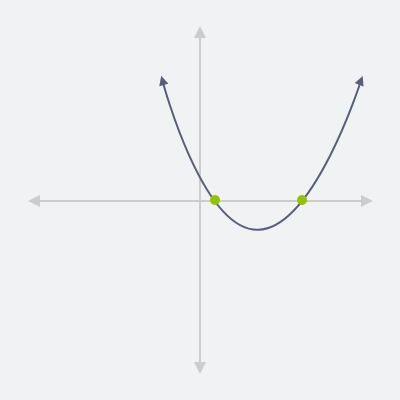If $$D = 0$$ -> Shows 1 repeated rootsIf $$D < 0$$ -> Shows no real roots## FAQS

Q1: What is discriminant?

Answer: When solving with the quadratic formula the “discriminant” is the value that helps us figure out the types of solutions, it reveal information about the roots of the equations.
It is represented by $$Δ$$ (delta).

Q2: What is the symbol of discriminant?

Answer: It is represented by D or $$Δ$$

Q3: What is the formula of discriminant?

Answer: $$Δ = b^2 – 4ac$$

Q4: Why do we find the discriminant?

Answer: By knowing the values of $$Δ$$, we can decide, whether we go further or not, as if it is negative so there are no real solutions.

Q5: What does $$Δ$$ predicts?

Answer: The $$Δ$$ (determinant) tell us whether there are two solutions, one solution, or no solution.

Q6: What is the important of the determinant?

Answer: The determinant (Δ) determine the nature of the roots of the given quadratic equation, this data/information is very useful as it provides us an opportunity of double check.

Q7: How can we identify, the equation has real solutions?

Answer: On the basis of discriminant (Δ) $$Δ = b^2 – 4ac$$ sign we can easily assess how many real number solutions the quadratic equation has.

Q8: What the discriminants (Δ) tell us?

Answer: The discriminant tells us whether there are two solution, one solutions or no solution.

Q9: What does it mean if the discriminant is less than 0?

Answer: In this case function has no real roots therefore the parabola does not intersect the x-axis.

Q10: How many solutions are possible if discriminant is greater than zero?

Answer: Two solution (2 real solution) if perfect square; 2 rational roots if not perfect square; 2 irrational roots.

• Embed Calculator Widget
• Direct URL